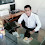### My Shameful Math Secret

One of these things is not like the others...The Papa                      L1                        L2                       L3Me

I LOVE math!  Third grade math.  I would teach it all day if I could.  But when I get home... I live in a house full of math geeks.  Yes, real ones.  As in, people who understand the mathematical gobbledygook that makes the eyes of normal humans glaze over...  My husband has been a math teacher.  His favorite class to teach was Trigonometry.  My oldest son is in the process of becoming a High School math teacher.  My daughter is getting her Master's Degree in Mathematics.  My youngest son has other aspirations (thank goodness), but would be capable of teaching math someday as well.

And then there's me.  I feel like Charlie Brown in this Peanuts comic strip:Courtesy of Peanuts.com
Here's what a conversation looks like at my house:

Son#1:$f(x) = \sum_{n=0}^\infty a_n \left( x-c \right)^n = a_0 + a_1 (x-c)^1 + a_2 (x-c)^2 + a_3 (x-c)^3 + \cdots$
Daughter:
"That's true, but I would add the function f given by f(x)=(1 + x)α"

Son#2:
"Blah blah blah convergence, blah blah blah hyperbolas"

Husband:$f(a)+\frac {f'(a)}{1!} (x-a)+ \frac{f''(a)}{2!} (x-a)^2+\frac{f^{(3)}(a)}{3!}(x-a)^3+ \cdots.$
Me:
"I taught my third graders how to remember 7 x 8 = 56 today."

So here's that shameful secret: I don't understand anything they are saying! One of these things is not like the others. Sigh. I guess we all have our gifts. I will just be the very best teacher of multiplication facts that I can be. Who knows? Maybe some of my students will grow up to be math geeks! And I can say I helped build the foundation. =)

Happy Math Teaching, whatever kind!

1.2.